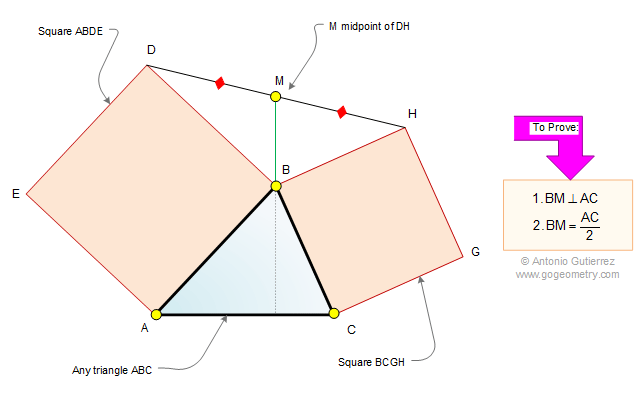Problem 502. Triangle, Two Squares, Midpoint, Perpendicular, Half the measure. Level: High School, College, SAT Prep. The drawing shows a triangle ABC with the squares ABDE and BCGH. If M is the midpoint of DH, prove that (1) BM is perpendicular to AC and (2) BM is half the measure of AC.Recent Additions